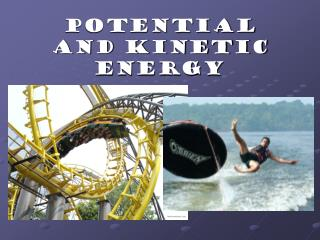DownloadDownload PresentationPotential and Kinetic Energy

# Potential and Kinetic Energy

Download Presentation## Potential and Kinetic Energy

- - - - - - - - - - - - - - - - - - - - - - - - - - - E N D - - - - - - - - - - - - - - - - - - - - - - - - - - -
##### Presentation Transcript

1. Potential and Kinetic Energy

2. Work and Energy • Work = force x distance • Work is only done when an object changes position • Expressed in joules • Energy = the ability to do work • Energy can be present in any object at any time • Can only be observed when energy is being transferred from one object/system to another • Amount of energy can be measured by how much work is done • Expressed in joules

3. Kinetic Energy Potential Energy Elastic Potential Energy Gravitation Potential Energy Chemical Potential Energy How is all energy divided? All Energy

4. What is Potential Energy? • Energy that is stored and waiting to be used later

5. What is Gravitational Potential Energy? • Potential energy due to an object’s position • P.E. = mass x gravity x height • PE = mgh

6. What is Elastic Potential Energy? • Potential energy due compression or expansion of an elastic object. Notice the ball compressing and expanding

7. More Examples of Elastic Potential Energy

8. What is Chemical Potential Energy? • Potential energy stored within the chemical bonds of an object

9. Another Example of Chemical Potential Energy

10. What is Kinetic Energy? KE = ½ mv2 • Energy an object has due to its motion • K.E. = .5 x mass x speed2

11. Law of Conservation of Energy Energy cannot be created or destroyed Energy changes form It’s transferred Total energy in a system remains the same

12. Energy Systems Closed – energy being transferred in and out is minimal (can be ignored) Open – exchange energy with space around them

13. Energy Transfer • KE is transferred into PE • PE is transferred into KE

14. Energy Transfer Notice that the “total” energy in the system stays the same

15. Wylie Coyote and Roderunner Cartoons

16. You try! http://www.eduplace.com/kids/hmsc/activities/simulations/gr4/unitf.html